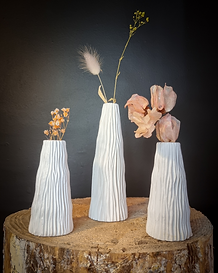top of pageTulip   Vase and Votive

Small model:

Dimensions: H = 7  cm  ; D  =  5  cm

Rate :   19 €

Big model :

Dimensions: H = 15.5  cm  ; D  =  9cm

Rate :   57€Ark Vases

Small model:

Dimensions: H=18  cm  ; D=  5.5cm

Rate :   39 €

Big model :

Dimensions: H = 19  cm  ; D  =  9.5  cm

Rate :   75 €Vayam and   Have

Vayam:

Dimensions: H = 22     cm  ; D   =  7   cm

Rate :   39

Have:

Dimensions: H = 26   cm  ; D   =  4.5   cm

Rate :   42Korall Vase

Small model:

Dimensions: H = 11.5   cm  ; D=   13.5   cm

Rate :   57 €

Big model :

Dimensions: H = 17cm  ; D   =  8cm

Rate :   49€Vaya

Small model:

Dimensions: D = 7.5  cm

Rate :   19 €

Big model :

Dimensions: H = 12.5  cm  ; D  =  17  cm

Rate :   69 €Virvel & Bolger

Virvel:

Dimensions: H = 14.5cm  ; D  =  12.5  cm

Rate :   49 €

Bolger:

Dimensions: H = 10  cm  ; D  =  10.5  cm

Rate :   29€Korall Soliflor

Small model:

Dimensions: H = 11   cm  ; D   = 5.5   cm

Rate :   24 €

Big model :

Dimensions: H = 16.5   cm  ; D   =  5.5   cm

Rate :   29 €Laly Hurricane

Small model:

Dimensions: H = 6.5  cm  ; D  = 7   cm

Rate :   21 €

Big model :

Dimensions: H = 15  cm  ; H=  13  cm

Rate :   75 €Bolger

Dimensions: H = 15  cm  ; D  =  14.5  cm

Rate :   49 €

bottom of page